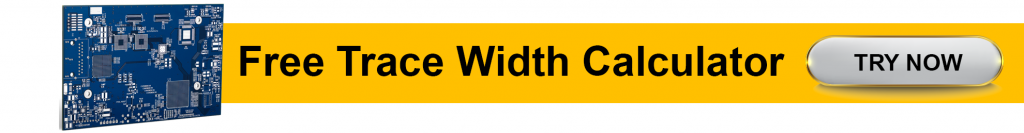# S-parameters Measurement Using a Vector Network Analyzer

by | Jun 28, 2021 | 0 comments

Designing a board for operating frequencies higher than a few hundred MHz becomes challenging as it is difficult to measure the voltage, current, or impedance of the circuit directly. This is where S-parameters come into play, as circuit/network parameters can be measured in terms of reflection and transmission coefficients. S-parameters deal with the transmission and reflection of signals for any network.

## Signal integrity

Signal integrity (SI) indicates the signal’s ability to propagate along PCB traces without distortion. Signal integrity is the measure of the quality of the signal (signal degradation) that passes through a transmission line from a driver to a receiver. While signal integrity is not a critical issue at lower frequencies, at higher frequencies (>50MHz) it plays a vital role. When it comes to high-speed boards, designers need to pay attention to both analog and digital aspects of the signal.

## Instrumentation to measure signal integrity

In the constantly evolving computing field, the shift from parallel to high-speed serial-data transmission is giving rise to new PCB design challenges. Data rates are increasing, pushing more bits per unit time through a single interconnect link/channel. These data rates have been nearing the Gigabit-per-second regime, leading to tighter timing budgets.

A symbol can be classified as either a pulse in digital baseband transmission or a tone in passband transmission using modems in telecommunication. It is can also be described as a waveform, a state or significant condition in the communication channel that persists for a fixed period.

Intersymbol interference (ISI) is a type of signal distortion in which one symbol interferes with consecutive symbols. This is an undesired event as the previous symbols have a similar effect as noise, affecting the reliability of the communication. Due to high-frequency interconnect losses, a higher data rate will lead to higher ISI. Another important consideration is higher data transmission rates requiring multiple serial links to work in parallel, generating multilane configurations. This will lead to crosstalk issues.

This is why you need to manage the characterization of channel reflections, losses, and crosstalk. Such characterization needs to be done in the frequency domain rather than the time domain using S or scattering parameters.

Two fundamental measurement methods are available for signal integrity characterization of channels in digital systems – time domain reflectometry (TDR) and vector network analyzer (VNA). To understand more about signal integrity download our SI design guide.

SIGNAL INTEGRITY FOR PCB DESIGNERS EBOOK### What is a time-domain reflectometer?

The time-domain reflectometer is an electronic device that uses reflected waveforms to decipher the characteristic impedance of PCB traces, a cable, a connector, and so on. It checks for electrical discontinuities in boards, connectors, or other transmission media.

In the early days of signal integrity measurement, the TDR was used to display the reflection coefficient or impedance versus distance. The location and nature of the electrical discontinuity can be precisely determined based on the timing, phase, and amplitude of the reflected waveform. TDR translates the reflected waveforms to generate a graphical representation of discontinuities present in a transmission channel.### What is a vector network analyzer?

A VNA includes a source that is used to create a known stimulus signal and a single or group of receivers that are used to detect changes to the said stimulus signal caused by the device-under-test or DUT. A DUT is a manufactured product that is subjected to testing, either right after manufacture or later during its life cycle as part of ongoing functional testing and calibration checks. The receivers of the vector network analyzer evaluate the resulting signals and compare them to the predetermined stimulus signal. It basically measures a component’s frequency response or a network made of several components, which can be both passive and active.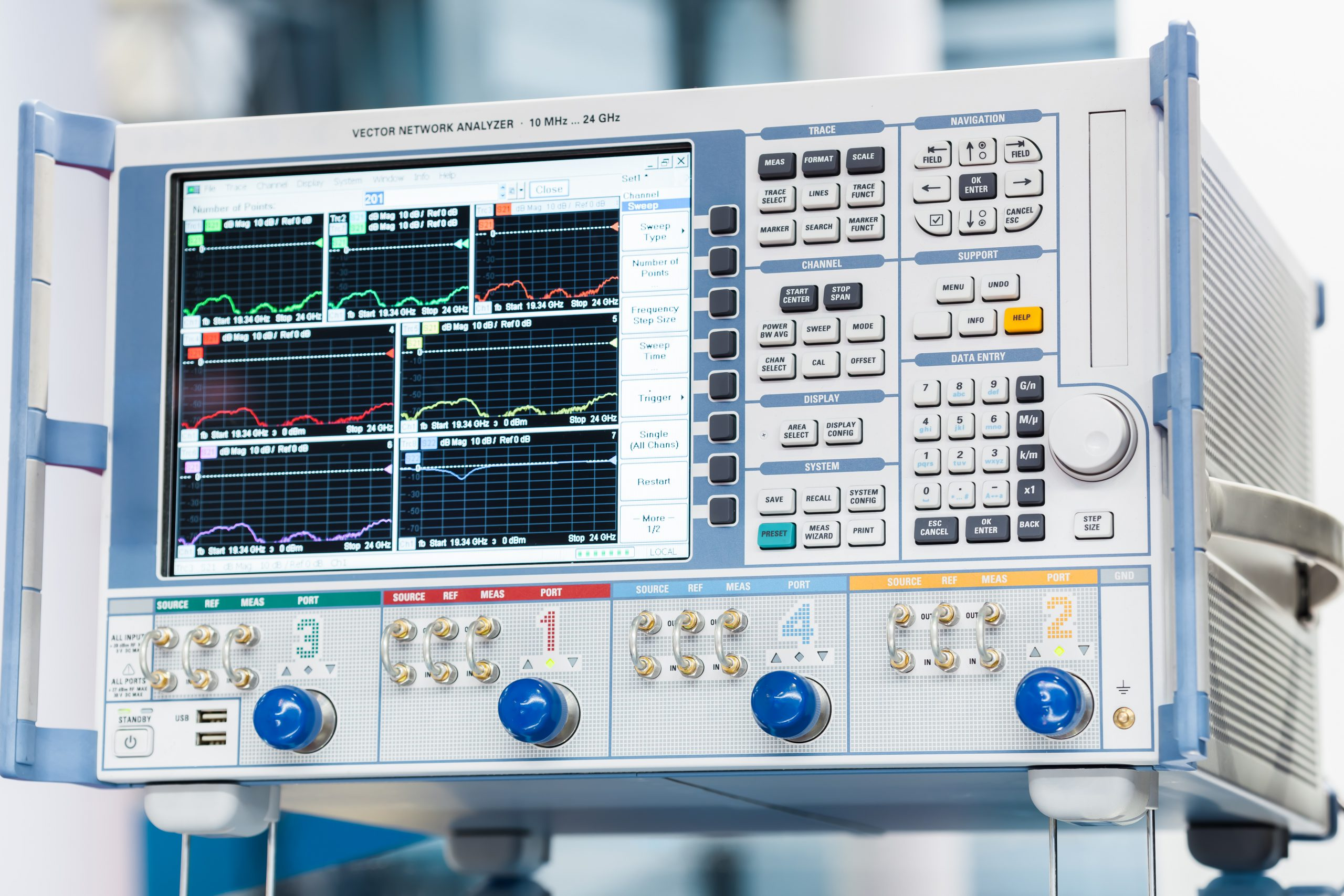Vector network analyzer for S-parameter measurement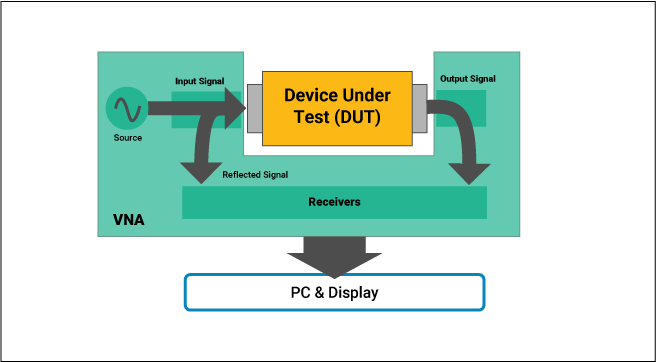Measurement of signal reflection caused by DUT in a VNA

A VNA basically measures the power of a high-speed signal that goes into and comes out of the component or network. This is because power, when compared to voltage and current, can be measured accurately at high frequencies. VNAs capture both the amplitude and phase of the signal at every point.

## What are S-parameters?

S-parameters are used to detail how energy can propagate through an electrical network. Scattering parameters are utilized to describe a complex network as a simple black box, analyzing the voltage and currents only at the input and output ports. It is particularly used to describe a network in terms of amplitude and phase vs. frequency.

S-parameters offer quantitive comprehension into the causes behind BER (bit error rate), ground bounce, jitter, and EMI. Also, you can measure crosstalk using scattering parameters to characterize signal transfer among adjacent transmission line pairs. Various electrical standards like 10GbE (10-Gbps Ethernet), PCIe (peripheral component interconnect express), SATA (serial advanced technology attachment), and fiber channels all use S-parameters in their compliance test procedures.

The blanket term SDNA (serial-data-network analysis) details testing for differential serial-data-compliance and for characterization of differential serial-data components.

Conventional tools for measuring S-parameters include VNAs. This equipment needs extensive calibration procedures. For SDNA uses, the aforementioned calibration processes can be really lengthy and complex leading to longer test times and susceptibility to human error. While electronic calibration modules for most SDNA applications are available, they operate at frequencies that are low for such applications. VNAs also cost more than other instrumentation for time-domain reflectometry but are more accurate.

## One-port network theory

In electrical circuits, a port includes a pair of terminals connecting an electrical circuit or network to an external device or a circuit. The external device will act as a point of entry or exit for electrical energy. Ports have to satisfy the condition that currents flowing into the two nodes need to be equal and in opposite directions.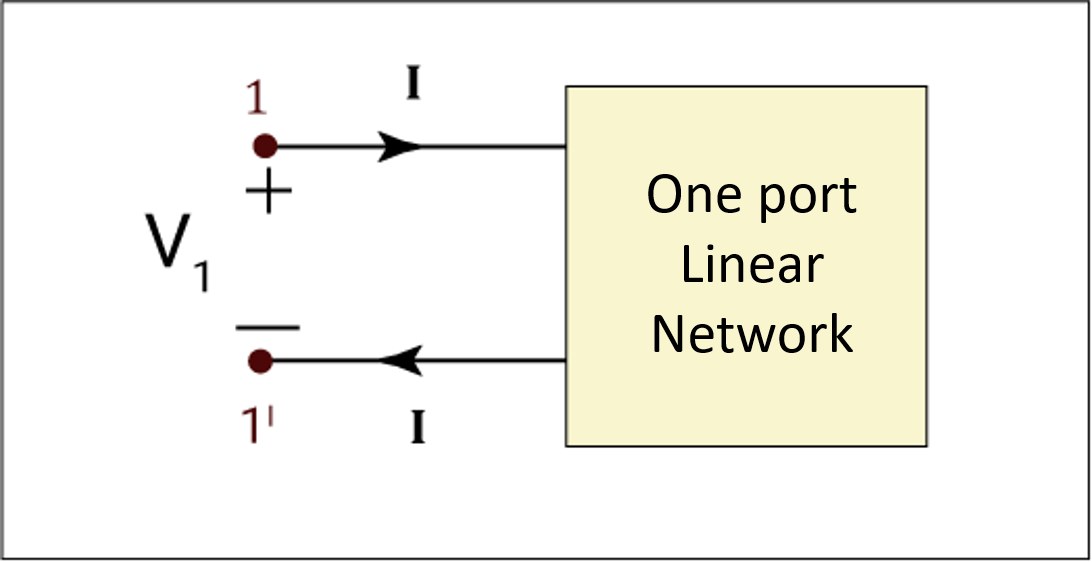One port, two-terminal network

A 1-port DUT has one S-parameter (S11). It is the ratio of the output voltage of Port 1 to the voltage going into Port 1. It is also known as return loss (RL) as a measure of reflected energy out of Port 1.

## Two-port network theory

Two-port network theory is a circuit analysis technique to describe a circuit with reference to two terminals by considering a circuit as a two-port network. In most electrical networks, obtaining the voltages and currents at the input and output ports is more convenient and practical than at any defined point in the circuit. The basic methodology here is that only the terminal variables such as input current/voltage and output current/voltage are considered for analysis. The voltages and currents within or inside the circuit are not considered here.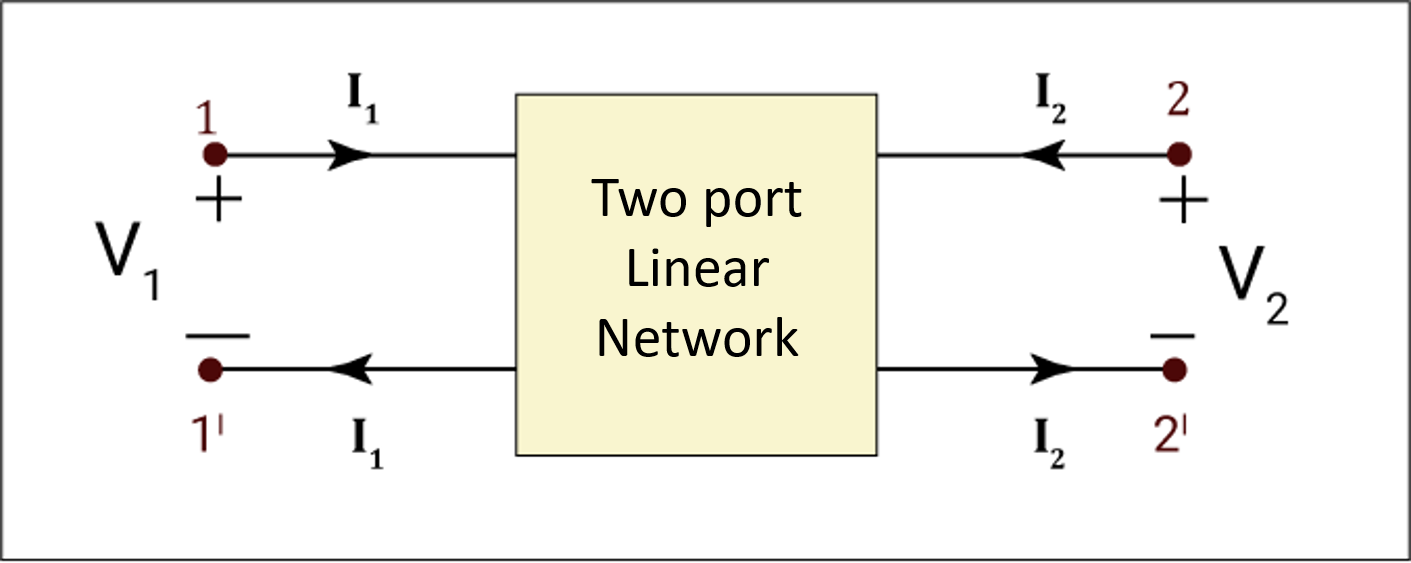Two-port, four-terminal network

In other network analysis techniques such as the use of Kirchhoff’s laws, or superposition theorem, one can calculate voltages and currents at any point in the circuit. Thevenin’s equivalent and Norton’s theorem enable one to obtain an equivalent circuit with regard to specified terminals in the network.

## What are S11 and S21?

S11 and S21 are input reflection and forward transmission coefficients respectively in a two-port network.

### Reflection Coefficient (S11)

The reflection coefficient is a quantity that describes how much of a wave is reflected by an impedance discontinuity in the channel of transmission. It can be defined as the ratio of the amplitude of the reflected wave to the incident wave.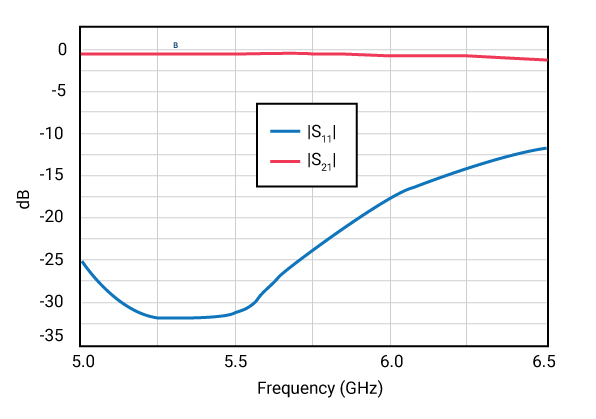Scattering parameters S11 and S21 in magnitude vs. frequency plot

The graph above shows a generic interpretation of a frequency response curve generated by vector network analyzers.

### Transmission coefficient (S21)

The transmission coefficient (S21) tells us how the transmission line transmits the signal. It is related to the insertion loss in the transmission.

S11 and S21 are calculated as follows: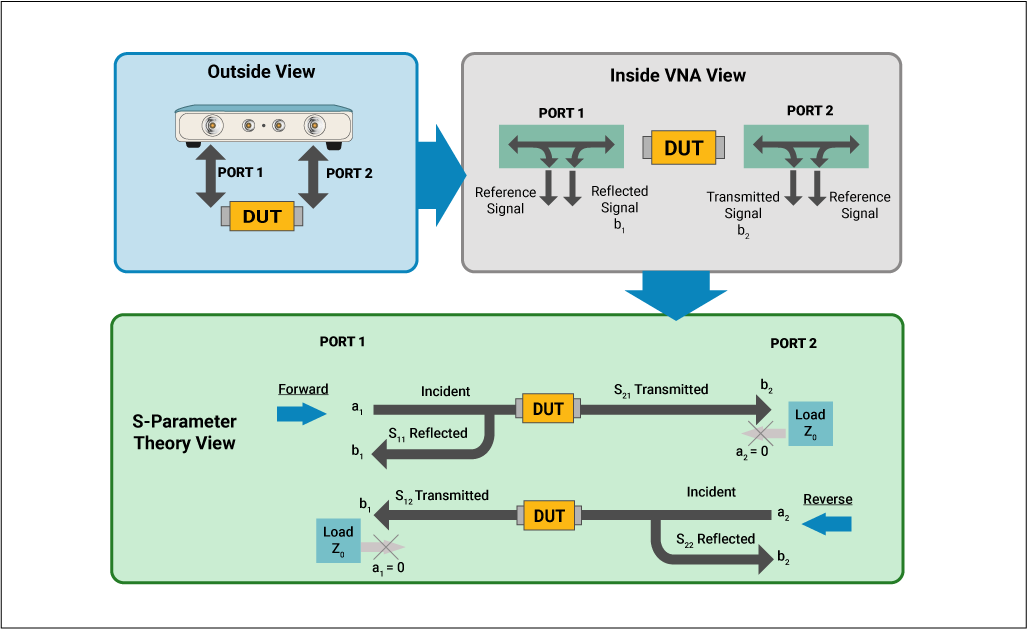Theoretical depiction of S-parameters using a VNA

a1 = traveling wave incident on port 1

a2 = traveling wave incident on port 2

b1 = traveling wave reflected from port 1

b2 = traveling wave reflected from port 2

A 2-port DUT has four S-parameters:

S11 = b1/a1 =  the input reflection coefficient.

S12 = b2/a2 = the reverse transmission coefficient.

S21 = b2/a1 = the forward transmission coefficient.

S22 = b1/a2 = the reverse reflection coefficient.

## Reflections on transmission lines

A signal that travels along an electrical transmission line will tend to be partially, or completely reflected back in the opposite direction when the signal meets a discontinuity in the line’s characteristic impedance. Reflections also result when the far end of the transmission line is not terminated in its characteristic impedance. They can also occur when two line lengths of different transmission lines are joined together.

The reflections on transmission lines are measured as the ratio of the reflected signal to the incident signal. Network analyzers are used to conduct reflection measurements, measuring the incident and reflected signal.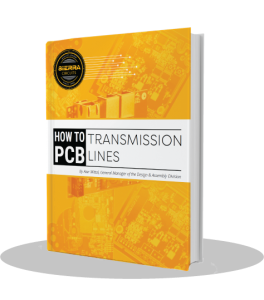### Return Loss

The simplest method to measure reflection is return loss. Return loss measures how much reflected power is lost when compared to an open, using the DUT. This parameter is expressed in decibel (dB) and it is a scalar (amplitude only) quantity. A really well-matched DUT will cause a massive loss in the power reflected from the open which leads to a higher return loss. With increasing reflections, a badly matched DUT will have a much lower loss in reflected power compared to an open, making its return loss really small.Transmission and reflection of power through a component

Insertion Loss = incident power/ transmitted power

Return Loss = incident power/ reflected power

In logarithmic terms, the formula for return loss is given as: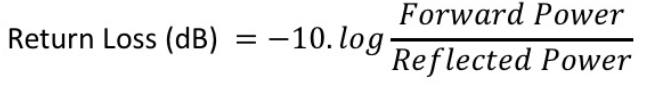Even if the measured reflection value on the VNA is -25dB, the negative sign is ignored when representing return loss. The component is said to have a loss of 25dB.

### Voltage standing wave ratio (VSWR)

Two waves traveling in opposite directions on the same transmission line cause a “standing wave” if they have the same amplitude and frequency. This occurrence can be measured in terms of the voltage standing wave ratio (VSWR). VSWR is defined as the ratio of the maximum reflected voltage to the minimum reflected voltage at a specified frequency. VSWR is a scalar quantity that provides only the amplitude. This parameter varies between one for a perfect match and infinity for an open or short circuit or lossless reactance.

## What is the difference between small-signal and large-signal S-parameters?

The main difference between small-signal and large-signal S-parameter is that the former deals with linear effects on a network or circuit whereas the latter deals with non-linear effects on a network or circuit. We will now discuss in detail what is meant by these two parameters.

### Small-signal S-parameters

Small signals in this scenario refer to signals that only have linear effects on the network. The signal will qualify as small-signal when gain compression or other non-linear effects do not take place. Gain compression is said to take place when an amplifier’s input power is increased to a level that decreases the amplifier gain, causing a non-linear increase in output power.

Small-signal S-parameters are defined as the ratio of reflected and incident waves.

When it comes to passive networks, small-signal is the only concern in terms of signal behavior as they act linearly at all power levels.

### Large-signal S-parameters

Large signals deal with non-linear circuits, and large-signal S-parameters are based on a harmonic balance simulation of the full non-linear circuit. Harmonic balance is a frequency-domain analysis method used for the simulation of distortion in non-linear circuits and systems.

Large-signal circuit simulation methods include non-linear effects such as gain compression. This leads to a change in scattering parameters when power levels are varied. It is also why large-signal S-parameters are called power-dependent S-parameters. Similar to small-signal S-parameters, large-signal scattering parameters are described as the ratio of reflected and incident waves.

### Mixed-mode S-parameters

Signal integrity engineers often have to check transmission line models and S-parameter measurements against frequency vs. gain compression plots (cartesian coordinates) or Smith charts (polar coordinates). Most such plots are expressed in mixed-mode S-parameters, meaning that the single-ended measurements require to be transformed to a mixed-mode matrix.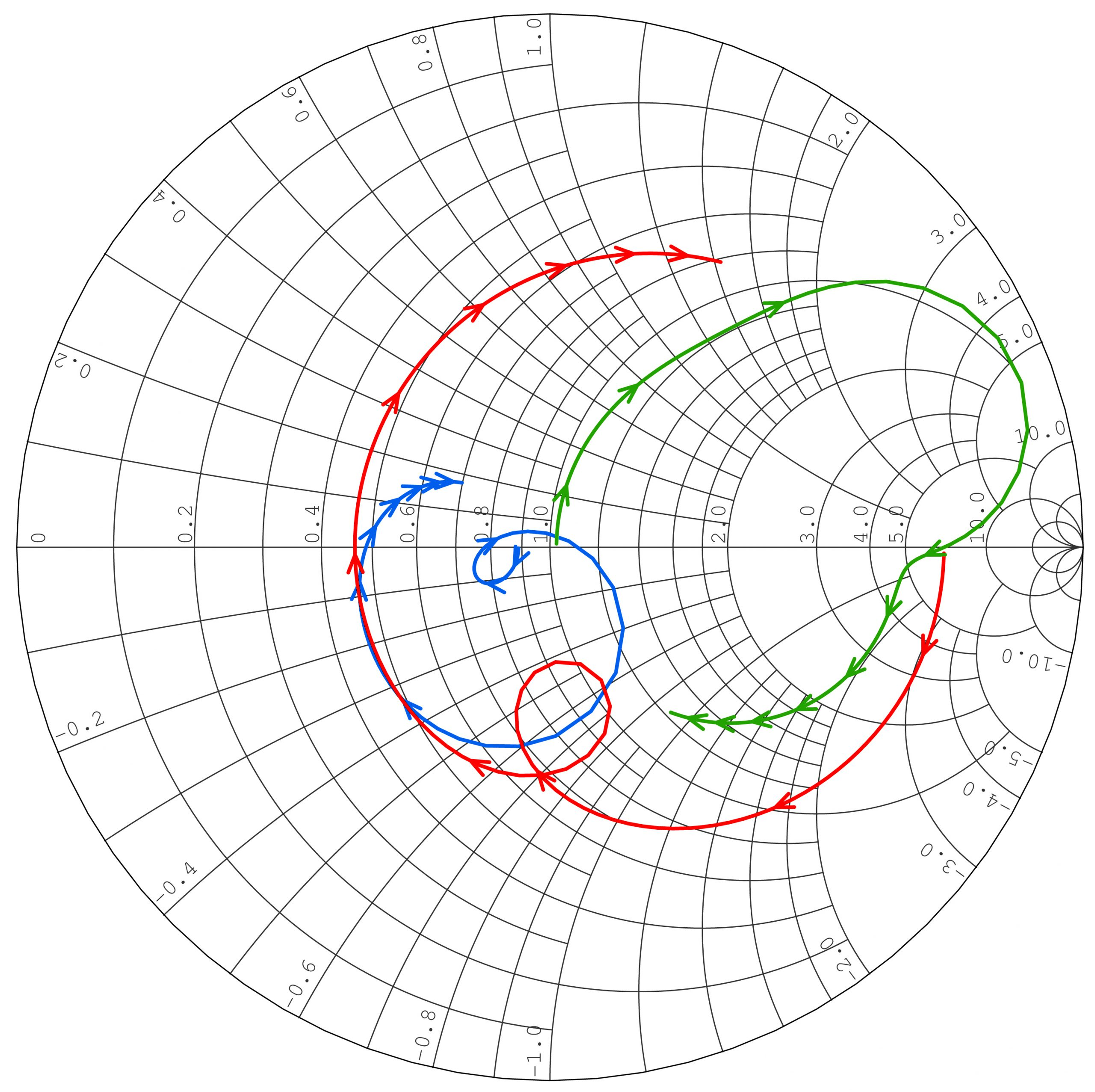Smith chart with S-parameters (red, green, and blue curves)

Two single-ended transmission lines with coupling are also known as a differential pair of transmission lines. When we talk about single-ended transmission lines with coupling, we are usually interested in characteristic impedance (Zo), phase delay, and NEXT/FEXT (near-end crosstalk and far-end cross talk) relationships.

S-parameters enable engineers to analyze complex networks’ behavior with relative ease, helping them accurately predict their response to signals on any of its inputs. This aids in the design of efficient controlled impedance PCBs.

Let us know in the comments if you want to learn something specific to S-parameters. We will be happy to address your queries.